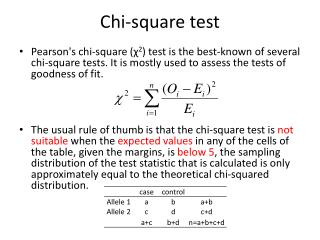DownloadDownload PresentationChi-square test

# Chi-square test

Télécharger la présentation## Chi-square test

- - - - - - - - - - - - - - - - - - - - - - - - - - - E N D - - - - - - - - - - - - - - - - - - - - - - - - - - -
##### Presentation Transcript

1. Chi-square test • Pearson's chi-square (χ2) test is the best-known of several chi-square tests. It is mostly used to assess the tests of goodness of fit. • The usual rule of thumb is that the chi-square test is not suitable when the expected values in any of the cells of the table, given the margins, is below 5, the sampling distribution of the test statistic that is calculated is only approximately equal to the theoretical chi-squared distribution.

2. Genetic Models • Genotypic P value • Allelic P value a= the number of AA in cases; b=the number of AB in cases; c=the number of BB in cases d= the number of AA in controls; e=the number of AB in controls; c=the number of BB in controls

3. Genetic Models Multiplicative model: r-fold increased risk for AB, r2 increased risk for BB. Analyzed by allele, not by genotype Additive model: r-fold increased risk for AB, 2rincreased risk for BB. Genotypes analysed by Armitages’s test for trend

4. Genetic Models Dominant model: Allele B increases risk Recissive model: Two copies of allele B required for increased risk

5. Fisher’s exact test • Fisher's exact test is a statistical significance test used in the analysis of contingency tables where sample sizes are small. • P=

6. ODDs Ratio • The odds ratio is the ratio of the odds of an event occurring in one group to the odds of it occurring in another group, or to a sample-based estimate of that ratio. • Odds of allele A in Cases = a x (a + b)/ b x (a + b) = a / b • Odds of allele B in Controls = d x (c + d) / d x (c + d) = c / d • OR=(a  d)/(b  c)

7. ODDs Ratio Suppose B is the risk allele ORhomo = ORBB= (c d)/(a f) ORhetero = ORAB= (b d)/(a e)

8. Population Attributable Risk (PAR) Pe is proportion of exposure in general population. People usually take proportion of exposure in the controls as Pe. (PAR is the reduction in incidence that would be observed if the population were extirely unexposed compared with its current (actual) exposure pattern)

9. Permutation Test The term permutation is used with different but closely related meanings. They all relate to the notion of mapping the elements of a set to other elements of the same set, i.e., exchanging (or "permuting") elements of a set

10. Permutation Test

11. Permutation Test • Permutation procedures provide a computationally intensive approach to generating significance levels empirically. • In samples of unrelated individuals, one simply swaps labels to provide a new dataset sampled under the null hypothesis. • Because the permutation schemes preserve the correlational structure between SNPs, this provides a less stringent correction for multiple testing in comparison to the Bonferroni, which assumes all tests are independent.

12. Power of association study

13. Number of patients and controls required to have 80% or 90% power to detect an association with a two-sided P-value of 10-5 for various risk ratios and population frequency of an allele/haplotype